>

#### NCERT Solutions for Class 12th: Ch 3 Matrices Exercise 3.2 Math

Page No: 80

Exercise 3.2

Question: 1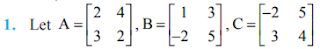Find each of the following:
(i) A + B
(ii) A – B
(iii) 3A – C
(iv) AB
(v) BA

(i) A + B(ii) A - B(iii) 3A – C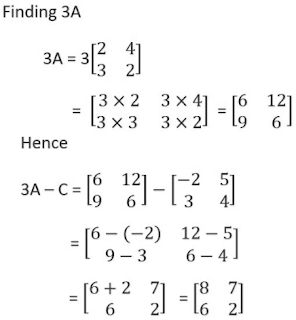(iv) AB(v) BA2. Compute the following:(i)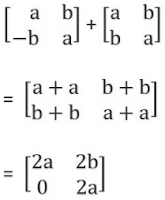(ii)(iii)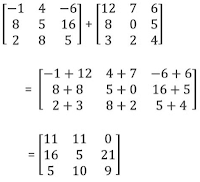(iv)3. Compute the indicated products.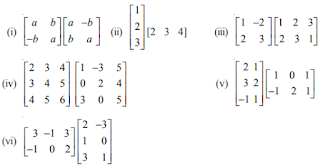(i)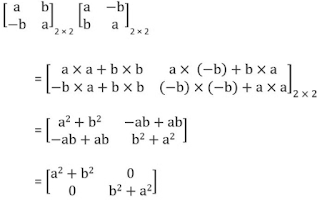(ii)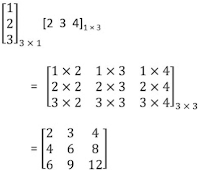(iii)(iv)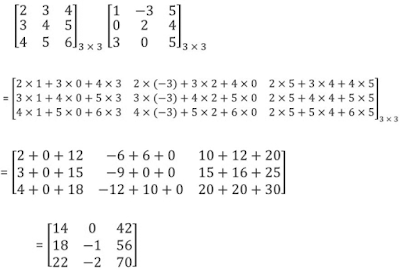(v)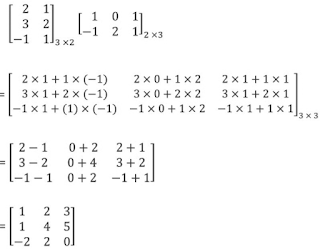(vi)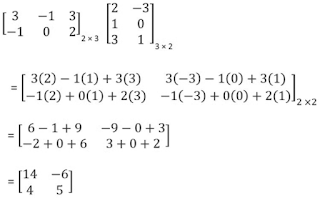Page No. 81

Question: 4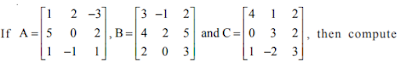(A+B) and (B – C). Also, verify that A + (B – C) = (A + B) – C.